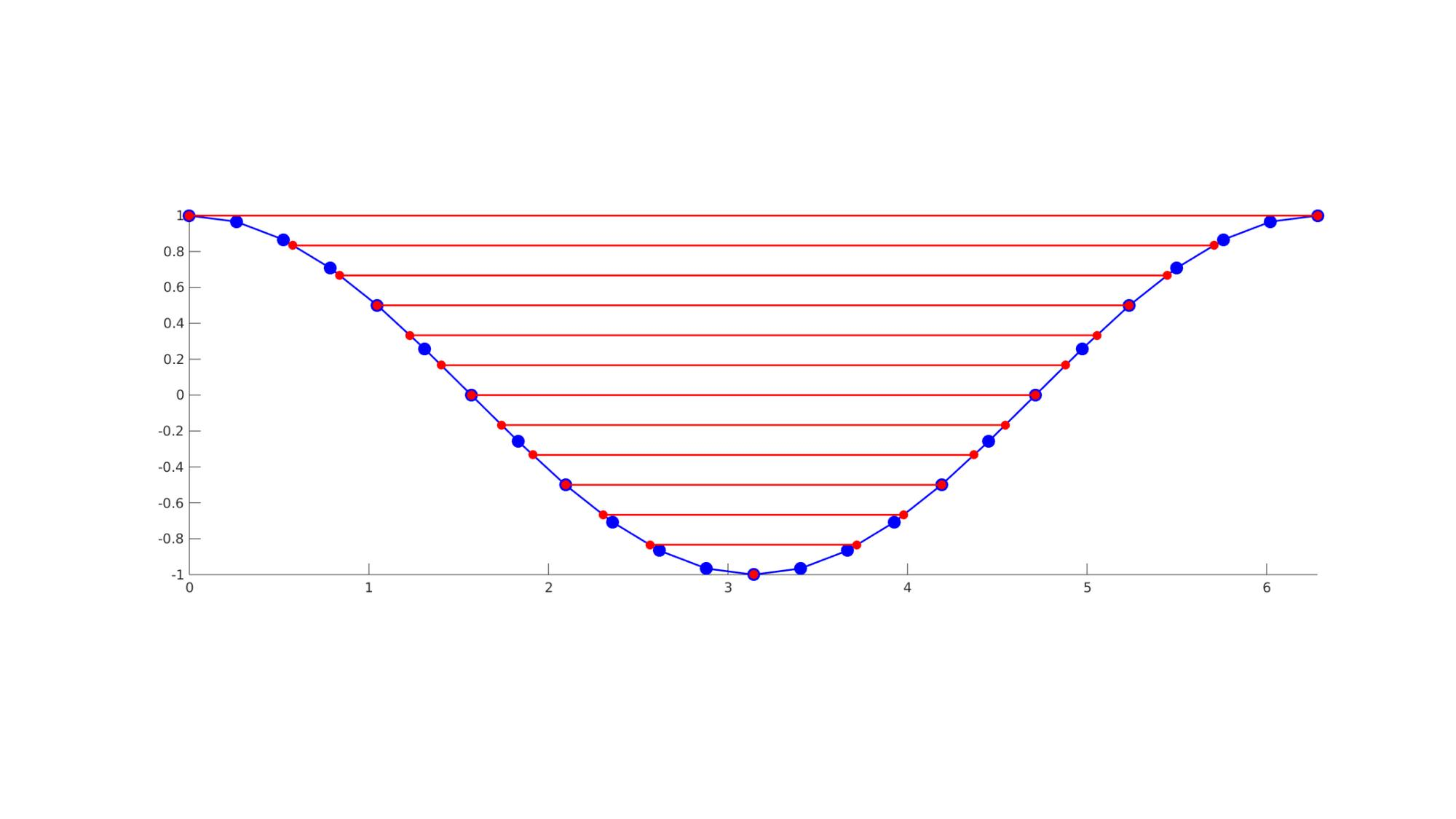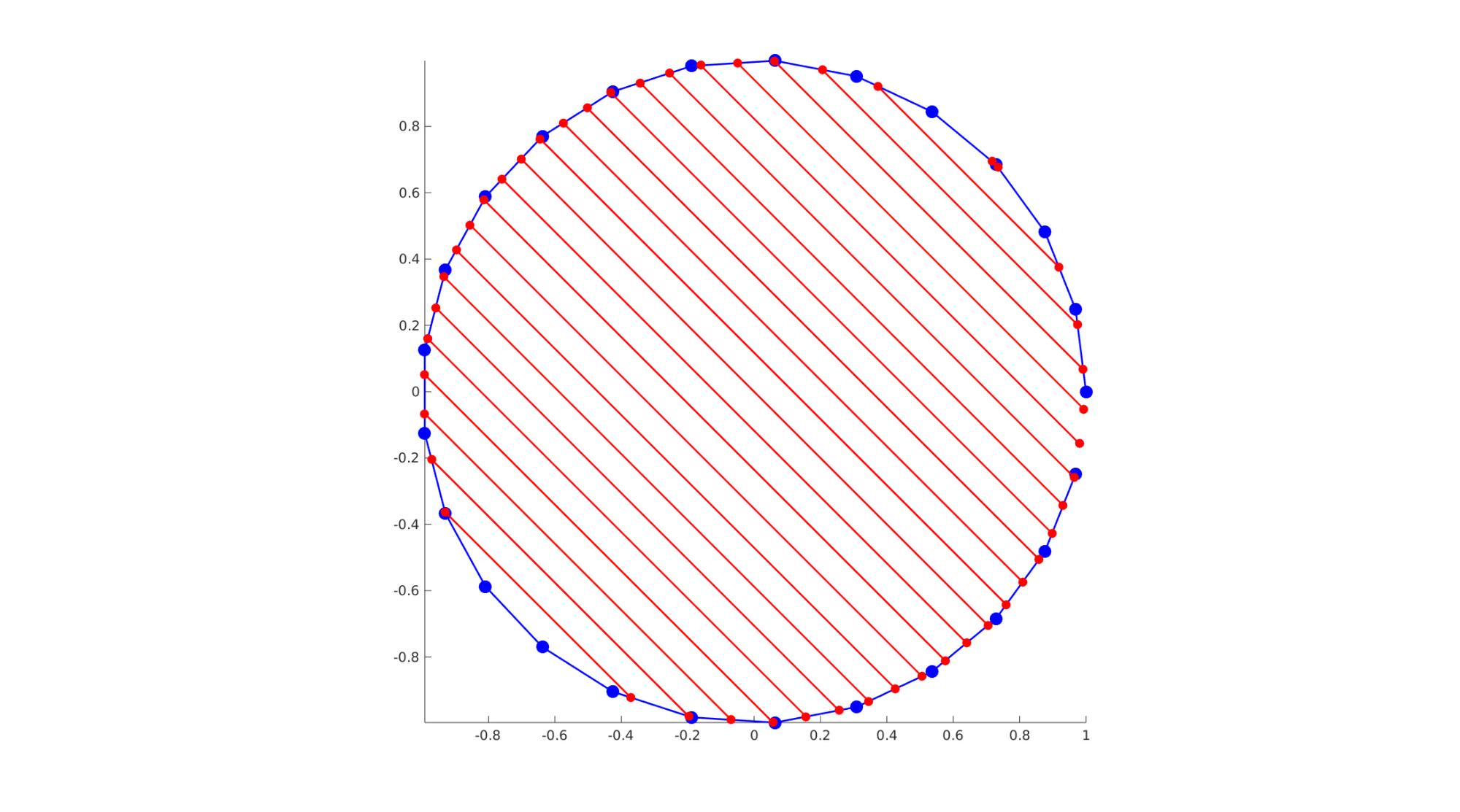# polyLineIntersect

Below is a demonstration of the features of the polyLineIntersect function

## Contents

```clear; close all; clc;
```

## Syntax

[Vn]=polyLineIntersect(V,n_cut,cutLevel,isClosed);

## Description

Computes the intersection points between a polygon defined by the vertex array V and a line defined the the normal vector n_cut and the cutLevel. If isClosed==1 (True) then the curve is assumed to be closed (additional line element from start to end is included.

PLOT SETTINGS

```fontSize=15;
lineWidth=2;
markerSize1=50;
markerSize2=35;
```

## Example 1: Computing intersections for a non-closed curve

```n=25;
t=linspace(0,2*pi,n)';

V_now=[t cos(t) zeros(size(t))];
n_cut=vecnormalize([0 1 0]);
isClosed=0;

numCutLevels=25;
cutLevel=linspace(-2,2,numCutLevels)';

cFigure; hold on;
plotV(V_now,'b.-','MarkerSize',markerSize1,'LineWidth',lineWidth);
axis tight; axis equal;
set(gca,'FontSize',fontSize);
drawnow;

for q=1:1:numel(cutLevel)

[Vn]=polyLineIntersect(V_now,n_cut,cutLevel(q),isClosed);

logicIntersect = any(~isnan(Vn),2);
if any(logicIntersect)
Vn_intersect = Vn(logicIntersect,:);
plotV(Vn_intersect,'r.-','MarkerSize',markerSize2,'LineWidth',lineWidth);
end
end
```## Example 2: Computing intersections for a closed curve

```n=25;
t=linspace(0,2*pi,n+1)'; t=t(1:end-1);

V_now=[cos(t) sin(t) zeros(size(t))];
n_cut=vecnormalize([1 1 0]);
isClosed=1;

numCutLevels=25;
cutLevel=linspace(max(V_now(:,2)),min(V_now(:,2)),numCutLevels)';

cFigure; hold on;
plotV(V_now,'b.-','MarkerSize',markerSize1,'LineWidth',lineWidth);
axis tight; axis equal;
set(gca,'FontSize',fontSize);
drawnow;

for q=1:1:numel(cutLevel)

[Vn]=polyLineIntersect(V_now,n_cut,cutLevel(q),isClosed);

logicIntersect = any(~isnan(Vn),2);
if any(logicIntersect)
Vn_intersect = Vn(logicIntersect,:);
plotV(Vn_intersect,'r.-','MarkerSize',markerSize2,'LineWidth',lineWidth);
end
end
```GIBBON www.gibboncode.org

Kevin Mattheus Moerman, [email protected]

GIBBON footer text

GIBBON: The Geometry and Image-based Bioengineering add-On. A toolbox for image segmentation, image-based modeling, meshing, and finite element analysis.

Copyright (C) 2006-2023 Kevin Mattheus Moerman and the GIBBON contributors

This program is free software: you can redistribute it and/or modify it under the terms of the GNU General Public License as published by the Free Software Foundation, either version 3 of the License, or (at your option) any later version.

This program is distributed in the hope that it will be useful, but WITHOUT ANY WARRANTY; without even the implied warranty of MERCHANTABILITY or FITNESS FOR A PARTICULAR PURPOSE. See the GNU General Public License for more details.

You should have received a copy of the GNU General Public License along with this program. If not, see http://www.gnu.org/licenses/.